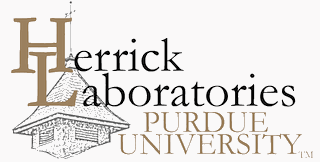## International Refrigeration and Air Conditioning Conference

2018

#### Keywords

CFD; simulation; forced-ventilation; condenser; 1d-3d-coupling

#### Abstract

To further improve the efficiency of refrigerators and freezers, it is necessary to optimize the whole cooling cycle. With one-dimensional numerical simulation programs, the transient behavior of the refrigeration cycle over more than 24 hours can be calculated. A major challenge here is to find appropriate heat transfer coefficients especially when the heat transfer is mainly influenced by forced-ventilation. In the present paper a refrigerator with a forced-air cooled condenser is investigated. To take the influences of the air flow and the condenser geometry on the simulated heat transfer into consideration, three-dimensional flow simulations are used. Due to superheating, subcooling and the transient behavior, the temperature varies across the condenser. Since the transferred heat strongly depends on the distribution of the temperature across the condenser surface, it would be necessary to run the 3d-CFD-simulation after every time step of the 1-d-simulation. To avoid this tremendous numerical effort, a more efficient method with so-called factors of influence is introduced. These factors of influence are calculated, using the results of around 30 CFD-simulations, which are performed with different temperature distributions. After the determination of these factors, the heat transfer for a given condenser geometry can be calculated for different temperature distributions with a simple algebraic equation. To validate this procedure, CFD-simulations with random temperature distributions are performed and compared with the results of the new method. The developed method has been implemented into the 1d-cycle-simulation. Thus it is possible to consider the geometry of the condenser and the complex flow field in the 1d-simulation without a noticeable increase of the computational effort. Finally the results of the cycle simulation are validated with measurement results.

COinS